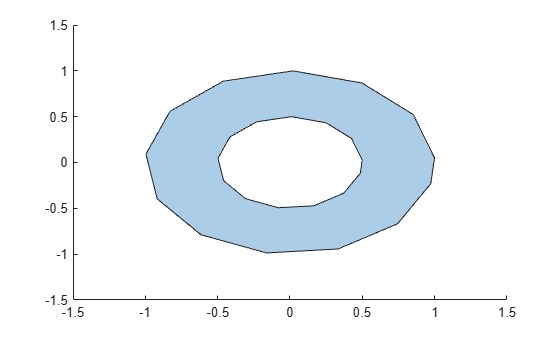ishole

Determine if polyshape boundary is a hole

Description

example

TF = ishole(polyin) returns a logical vector whose elements are 1 (true) if the corresponding boundary of polyin is a hole.

TF = ishole(polyin,I) returns a logical vector corresponding to the boundaries of polyin indexed by I. TF is the same length as I.

Examples

collapse all

Create a polygon containing one solid region and one hole, and determine which region is a hole.

t = 0.05:0.5:2*pi;
x1 = cos(t);
y1 = sin(t);
x2 = 0.5*cos(t);
y2 = 0.5*sin(t);
polyin = polyshape({x1,x2},{y1,y2})
polyin =
polyshape with properties:

Vertices: [27x2 double]
NumRegions: 1
NumHoles: 1

plot(polyin)TF = ishole(polyin)
TF = 2x1 logical array

0
1

To query one boundary at a time, use the boundary index as a second argument.

TF = ishole(polyin,2)
TF = logical
1

Input Arguments

collapse all

Input polyshape, specified as a scalar.

Data Types: polyshape

Boundary index, specified as a scalar integer or vector of integers. Each element of I corresponds to a single boundary of the input polyshape.

Data Types: double | single | int8 | int16 | int32 | int64 | uint8 | uint16 | uint32 | uint64